Contents >> Applied Mathematics >> Numerical Methods >> Numerical Differentiaon >> Formulas of numerical differentiaon

 Numerical differentiaon - Formulas of numerical differentiation Formulas of numerical differentiation 1. On a basis of Newton’s first interpolating formula For finding of the first and second derivatives of function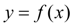, given in equidistant points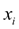( i = 0, 1, 2, …, n ) of segment [ a , b ] by values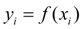, it is approximately replaced with Newton’s interpolating polynomial constructed for system of nodes: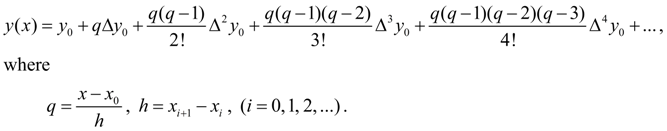(5) Removing brackets and considering, that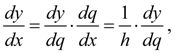we’ll receive: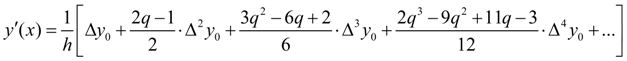.        (6) Similarly, considering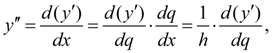we’ll receive: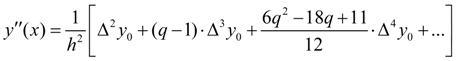.       (7) In the same way if necessary, it is possible to calculate any order derivative of function. We’ll notice, that at calculation of derivatives in fixed point х as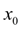it is necessary to take the nearest tabular value of argument. It is possible to deduce also formulas of numerical differentiation based on Newton’s second interpolating formula . 2. On a basis of Newton’s second interpolating formula Let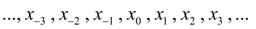– system of equidistant points with step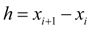and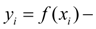corresponding values of given function. Putting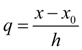and replacing the function by Stirling’s interpolating polynomial, we’ll receive: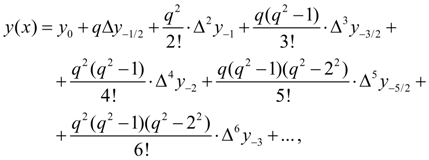(8) where for brevity of record the following designations are entered: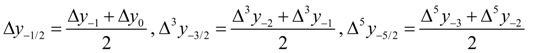and so on. From (8) in view of that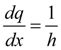, follows: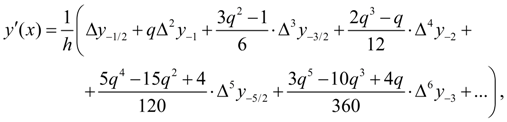(9)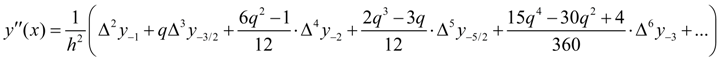. (10)

 < Previous Contents Next >